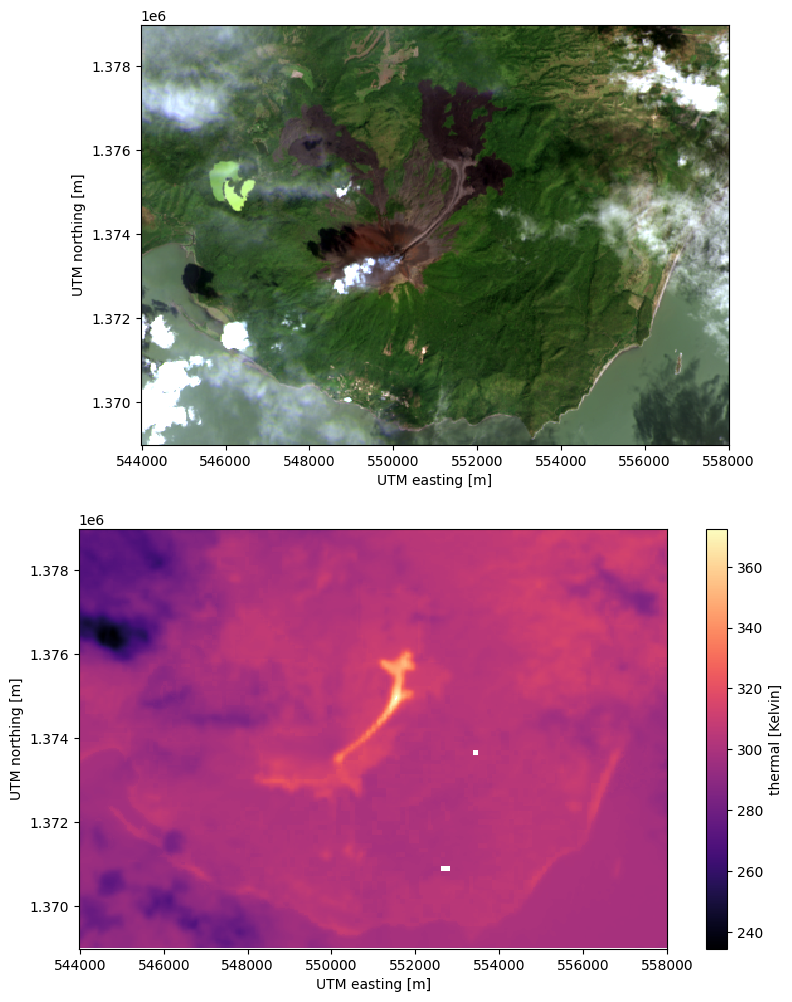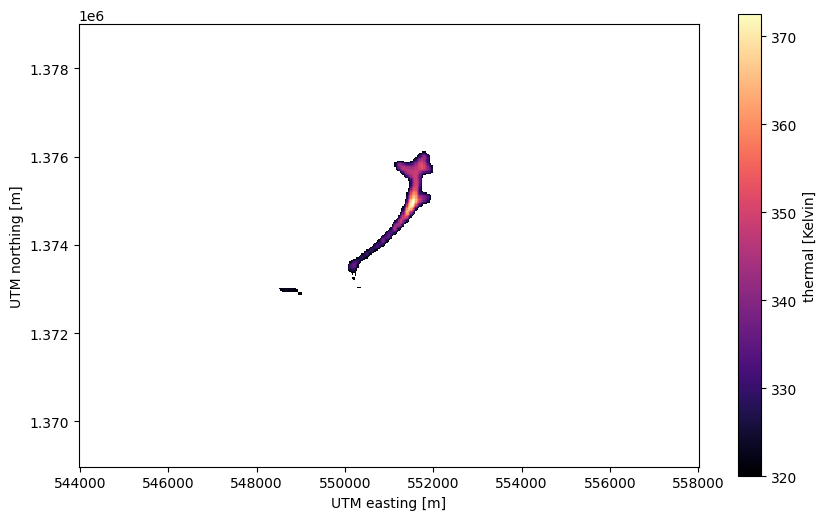# Plotting bands overlaid on composites#

Sometimes, we may want to overlay a part of a band (for example, the thermal band) on top of a composite. The overlay band can be partially transparent, cropped to a range of values, or both. As an example, let’s make a plot of the December 2015 eruption of Momotombo volcano, Nicaragua. We’ll overlay the thermal band (only pixels above 320 K) on top of an RGB composite to show the ongoing lava flow.

First, we’ll import the required packages and load the sample scene:

```import xlandsat as xls
import matplotlib.pyplot as plt
import xarray as xr
import numpy as np

path = xls.datasets.fetch_momotombo()
# Fill the missing values due to the volcanic clouds to make it look nicer
scene = xls.interpolate_missing(scene)
scene
```
```<xarray.Dataset>
Dimensions:   (easting: 467, northing: 333)
Coordinates:
* easting   (easting) float64 5.44e+05 5.44e+05 ... 5.58e+05 5.58e+05
* northing  (northing) float64 1.369e+06 1.369e+06 ... 1.379e+06 1.379e+06
Data variables:
blue      (northing, easting) float16 -0.05554 -0.05481 ... 0.07788 0.07727
green     (northing, easting) float16 0.04834 0.05066 ... 0.1056 0.1027
red       (northing, easting) float16 0.006226 0.01105 ... 0.1163 0.1045
nir       (northing, easting) float16 0.01483 0.02124 ... 0.2435 0.2367
swir1     (northing, easting) float16 0.06189 0.06409 ... 0.2098 0.1976
swir2     (northing, easting) float16 0.06519 0.06628 ... 0.1571 0.1527
thermal   (northing, easting) float16 293.5 293.5 293.8 ... 311.0 312.0
Attributes: (12/19)
Conventions:                CF-1.8
title:                      Landsat 8 scene from 2015-12-05 (path/row=17/51)
digital_object_identifier:  https://doi.org/10.5066/P9OGBGM6
origin:                     Image courtesy of the U.S. Geological Survey
landsat_product_id:         LC08_L2SP_017051_20151205_20200908_02_T1
processing_level:           L2SP
...                         ...
ellipsoid:                  WGS84
date_acquired:              2015-12-05
scene_center_time:          16:06:06.8773380Z
wrs_path:                   17
wrs_row:                    51
mtl_file:                   GROUP = LANDSAT_METADATA_FILE\n  GROUP = PROD...```

Now we can plot an RGB composite and thermal band separately to see that they have to show:

```# Make the composite
rgb = xls.composite(scene, rescale_to=(0, 0.6))

# Histogram equalization for a better looking image
rgb = xls.equalize_histogram(rgb, clip_limit=0.02, kernel_size=200)

# Plot the RGB and thermal separately
fig, (ax1, ax2) = plt.subplots(2, 1, figsize=(10, 12))

rgb.plot.imshow(ax=ax1)
scene.thermal.plot.imshow(ax=ax2, cmap="magma")

ax1.set_aspect("equal")
ax2.set_aspect("equal")

plt.show()
```Notice that the lava flow is clearly visible as temperatures above 320 K in the thermal band but it’s difficult to see where the volcano and other landmarks are. Looking at the RGB composite, we can’t really make out the lava flow but we have a clear picture of where the volcano is and where the old lava flows are. A way to show the thermal data with the geographic context of the RGB is to overlay the two in a single plot.

To do so, we’ll first create a version of the thermal band that has all pixels with temperature below 320 K set to NaN (not-a-number). This is used to indicate to matplotlib that a pixel should be transparent. An easy way to do this is with the `xarray.where` function:

```# If the condition is true, use the thermal values. If it's false, use nan
lava = xr.where(scene.thermal >= 320, scene.thermal, np.nan, keep_attrs=True)

fig, ax = plt.subplots(1, 1, figsize=(10, 6))

lava.plot.imshow(ax=ax, cmap="magma")

ax.set_aspect("equal")
plt.show()
```Note

We used the `keep_attrs=True` parameter to tell xarray that it should keep the metadata from the original band in the lava-only version. This will preserve the information on units, procedence, etc. But be careful with this since it can lead to metadata being propagated when it’s no longer valid.

Now that we have an `xarray.DataArray` with the lava flow only, we can plot that on top of the RGB composite and add a bit of transparency using the `alpha` parameter of `imshow`.

```fig, ax = plt.subplots(1, 1, figsize=(10, 6))

# RGB goes first so it's at the bottom
rgb.plot.imshow(ax=ax)
lava.plot.imshow(ax=ax, cmap="magma", alpha=0.6)

ax.set_aspect("equal")
plt.show()
```With the plot above, all of the information we have available about the lava flow is displayed in a nice format.